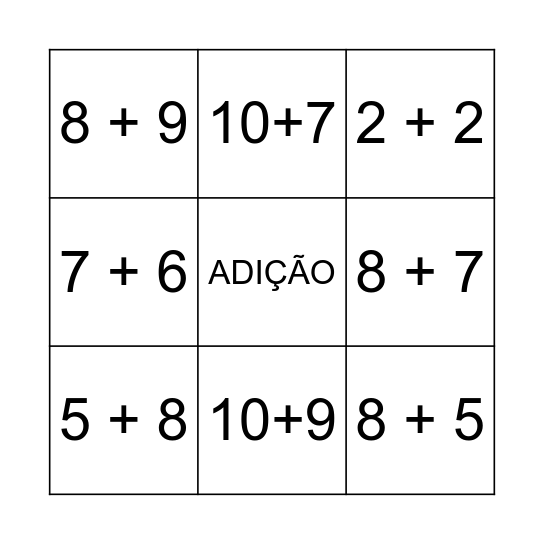# BINGOThis bingo card has a free space and 66 words: 10+2, 10+3, 10+4, 10+5, 10+1, 10+6, 10+7, 10+8, 10+9, 10+10, 8 + 2, 8 + 3, 8 + 4, 8 + 5, 8 + 6, 8 + 7, 8 + 8, 8 + 9, 7 + 2, 7 + 3, 7 + 4, 7 + 5, 7 + 6, 7 + 7, 7 + 8, 7 + 9, 6 + 2, 6 + 3, 6 + 4, 6 + 5, 6 + 6, 6 + 7, 6 + 8, 6 + 9, 5 + 2, 5 + 3, 5 + 4, 5 + 5, 5 + 6, 5 + 7, 5 + 8, 5 + 9, 4 + 2, 4 + 3, 4 + 4, 4 + 5, 4 + 6, 4 + 7, 4 + 8, 4 + 9, 3 + 2, 3 + 3, 3 + 4, 3 + 5, 3 + 6, 3 + 7, 3 + 8, 3 + 9, 2 + 2, 2 + 3, 2 + 4, 2 + 5, 2 + 6, 2 + 7, 2 + 8 and 2 + 9.

## Play Online